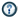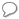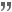xMathematicsOverview

Mathematics is the study of quantity
Quantity
Quantity is a property that can exist as a magnitude or multitude. Quantities can be compared in terms of "more" or "less" or "equal", or by assigning a numerical value in terms of a unit of measurement. Quantity is among the basic classes of things along with quality, substance, change, and relation...

, space
Space
Space is the boundless, three-dimensional extent in which objects and events occur and have relative position and direction. Physical space is often conceived in three linear dimensions, although modern physicists usually consider it, with time, to be part of a boundless four-dimensional continuum...

, structure
Structure
Structure is a fundamental, tangible or intangible notion referring to the recognition, observation, nature, and permanence of patterns and relationships of entities. This notion may itself be an object, such as a built structure, or an attribute, such as the structure of society...

, and change
Calculus
Calculus is a branch of mathematics focused on limits, functions, derivatives, integrals, and infinite series. This subject constitutes a major part of modern mathematics education. It has two major branches, differential calculus and integral calculus, which are related by the fundamental theorem...

. Mathematician
Mathematician
A mathematician is a person whose primary area of study is the field of mathematics. Mathematicians are concerned with quantity, structure, space, and change....

s seek out patterns and formulate new conjecture
Conjecture
A conjecture is a proposition that is unproven but is thought to be true and has not been disproven. Karl Popper pioneered the use of the term "conjecture" in scientific philosophy. Conjecture is contrasted by hypothesis , which is a testable statement based on accepted grounds...

s. Mathematicians resolve the truth or falsity of conjectures by mathematical proof
Mathematical proof
In mathematics, a proof is a convincing demonstration that some mathematical statement is necessarily true. Proofs are obtained from deductive reasoning, rather than from inductive or empirical arguments. That is, a proof must demonstrate that a statement is true in all cases, without a single...

s, which are arguments sufficient to convince other mathematicians of their validity. The research required to solve mathematical problems can take years or even centuries of sustained inquiry. However, mathematical proofs are less formal and painstaking than proofs in mathematical logic
Mathematical logic
Mathematical logic is a subfield of mathematics with close connections to foundations of mathematics, theoretical computer science and philosophical logic. The field includes both the mathematical study of logic and the applications of formal logic to other areas of mathematics...

.DiscussionsDefine Gausssian functionMathematical model of lumber drying processmathematicsQuotations

Mathematics is not a careful march down a well-cleared highway, but a journey into a strange wilderness, where the explorers often get lost. Rigour should be a signal to the historian that the maps have been made, and the real explorers have gone elsewhere.W.S. Anglin, in Mathematics and History, elucidating the symmetry between the creative and logical aspects of mathematics.

If in other sciences we should arrive at certainty without doubt and truth without error, it behooves us to place the foundations of knowledge in mathematics.Roger Bacon Opus Majus, bk.1, ch4.

Great fleas have little fleas upon their backs to bite 'em,And little fleas have lesser fleas, and so ad infinitum,And the great fleas themselves, in turn, have greater fleas to go on,While these again have greater still, and greater still, and so on.Augustus De Morgan, A Budget of Paradoxes

Numbers exist only in our minds. There is no physical entity that is number 1. If there were, 1 would be in a place of honor in some great museum of science, and past it would file a steady stream of mathematicians gazing at 1 in wonder and awe.Linear Algebra by Fraleigh/Beauregard

Euler calculated the force of the wheels necessary to raise the water in a reservoir … My mill was carried out geometrically and could not raise a drop of water fifty yards from the reservoir. Vanity of vanities! Vanity of geometry!Frederick II of Prussia|Frederick the Great, Letters of Voltaire and Frederick the Great (New York: Brentano's, 1927), transl. Richard Aldington, letter 221 from Frederick II of Prussia|Frederick to Voltaire, 25 November 1777.